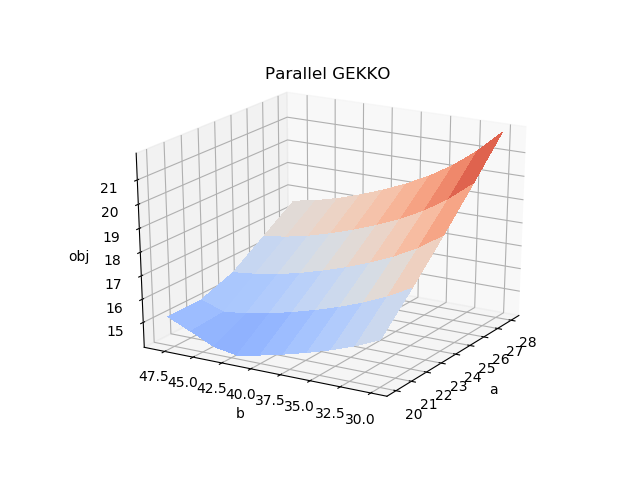### Parallel Computing in Optimization

Programs can run on multiple CPU cores or on heterogeneous networks and platforms with parallelization. In this example application, we solve a series of optimization problems using Linux and Windows servers using Python multi-threading. The optimization problems are initialized sequentially, computed in parallel, and returned asynchronously to the MATLAB or Python script.

In Python, parallelization is accomplished with multithreading. The following example shows an example of how to create and run a program with 10 threads that each print a message.

import datetime
import time, random

def __init__(self, id):
self.id = id
self.delay = random.random()
def run(self):
time.sleep(self.delay)
now = datetime.datetime.now()
print("ID => %s: %s completes at %s\n" % \
(self.id, self.getName(), now))

for i in range(10):

# Wait for all threads to complete
t.join()

The next step is to embed a simple Nonlinear Programming (NLP) problem into the multi-threaded application. The tutorial examples are available for download below:

import numpy as np
from gekko import GEKKO

# Optimize at mesh points
x = np.arange(20.0, 30.0, 2.0)
y = np.arange(30.0, 50.0, 4.0)
amg, bmg = np.meshgrid(x, y)

# Initialize results array
obj = np.empty_like(amg)

m = GEKKO(remote=False)
objective = float('NaN')

a,b = m.Array(m.FV,2)

# model variables, equations, objective
x1 = m.Var(1,lb=1,ub=5)
x2 = m.Var(5,lb=1,ub=5)
x3 = m.Var(5,lb=1,ub=5)
x4 = m.Var(1,lb=1,ub=5)
m.Equation(x1*x2*x3*x4>=a)
m.Equation(x1**2+x2**2+x3**2+x4**2==b)
m.Minimize(x1*x4*(x1+x2+x3)+x3)
m.options.SOLVER = 1 # APOPT solver

# Calculate obj at all meshgrid points
for i in range(amg.shape):
for j in range(bmg.shape):
a.MEAS = amg[i,j]
b.MEAS = bmg[i,j]

m.solve(disp=False)

obj[i,j] = m.options.OBJFCNVAL
print(amg[i,j],bmg[i,j],obj[i,j])

# plot 3D figure of results
from mpl_toolkits.mplot3d import Axes3D
import matplotlib.pyplot as plt
from matplotlib import cm
import numpy as np

fig = plt.figure()
ax = fig.gca(projection='3d')
surf = ax.plot_surface(amg, bmg, obj, \
rstride=1, cstride=1, cmap=cm.coolwarm, \
vmin = 10, vmax = 25, linewidth=0, antialiased=False)
ax.set_xlabel('a')
ax.set_ylabel('b')
ax.set_zlabel('obj')
plt.show()

import numpy as np
import time, random
from gekko import GEKKO

def __init__(self, id, server, ai, bi):
s = self
s.id = id
s.server = server
s.m = GEKKO()
s.a = ai
s.b = bi
s.objective = float('NaN')

# initialize variables
s.m.x1 = s.m.Var(1,lb=1,ub=5)
s.m.x2 = s.m.Var(5,lb=1,ub=5)
s.m.x3 = s.m.Var(5,lb=1,ub=5)
s.m.x4 = s.m.Var(1,lb=1,ub=5)

# Equations
s.m.Equation(s.m.x1*s.m.x2*s.m.x3*s.m.x4>=s.a)
s.m.Equation(s.m.x1**2+s.m.x2**2+s.m.x3**2+s.m.x4**2==s.b)

# Objective
s.m.Minimize(s.m.x1*s.m.x4*(s.m.x1+s.m.x2+s.m.x3)+s.m.x3)

# Set global options
s.m.options.IMODE = 3 # steady state optimization
s.m.options.SOLVER = 1 # APOPT solver

def run(self):

# Don't overload server by executing all scripts at once
sleep_time = random.random()
time.sleep(sleep_time)

print('Running application ' + str(self.id) + '\n')

# Solve
self.m.solve(disp=False)

# Results
#print('')
#print('Results')
#print('x1: ' + str(self.m.x1.value))
#print('x2: ' + str(self.m.x2.value))
#print('x3: ' + str(self.m.x3.value))
#print('x4: ' + str(self.m.x4.value))

# Retrieve objective if successful
if (self.m.options.APPSTATUS==1):
self.objective = self.m.options.objfcnval
else:
self.objective = float('NaN')
self.m.cleanup()

# Select server
server = 'https://byu.apmonitor.com'

# Optimize at mesh points
x = np.arange(20.0, 30.0, 2.0)
y = np.arange(30.0, 50.0, 2.0)
a, b = np.meshgrid(x, y)

# Calculate objective at all meshgrid points

id = 0
for i in range(a.shape):
for j in range(b.shape):
# Increment ID
id += 1

# Run applications simultaneously as multiple threads
# Max number of threads to run at once
time.sleep(0.01)
t.start()

# Check for completion
mt = 3.0 # max time
it = 0.0 # incrementing time
st = 1.0 # sleep time
time.sleep(st)
it = it + st
# Terminate after max time
if (it>=mt):
break

# Wait for all threads to complete
#    t.join()

# Initialize array for objective
obj = np.empty_like(a)

# Retrieve objective results
id = 0
for i in range(a.shape):
for j in range(b.shape):
id += 1

# plot 3D figure of results
from mpl_toolkits.mplot3d import Axes3D
import matplotlib.pyplot as plt
from matplotlib import cm
import numpy as np

fig = plt.figure()
ax = fig.gca(projection='3d')
surf = ax.plot_surface(a, b, obj, \
rstride=1, cstride=1, cmap=cm.coolwarm, \
vmin = 12, vmax = 22, linewidth=0, antialiased=False)
ax.set_xlabel('a')
ax.set_ylabel('b')
ax.set_zlabel('obj')